## Motivating Demo on CodePen

See the Pen AVL Tree w/ Insert + Delete in D3 by thmsdnnr (@thmsdnnr) on CodePen.

## Excellent Resources on AVL Trees

If you are a visual learner, these YouTube Lectures by Rob Edwards at San Diego State University are a fantastic resource. I highly recommend taking a look at them to get a sense of what rotations are doing before you dig too far into the code.

It’s key that you read the previous tutorial on AVL trees for this tutorial to make sense. We’ve covered the concepts of node balance factors, keeping node height differential to within `[-1, 1]`, and the use of rotations to correct imbalanced nodes.

Today we’re going to incorporate this balancing behavior into our tree structure so that the tree will automatically rebalance itself and update node height upon insertion and deletion.

## Generating Imbalance

Before we code, let’s first consider how trees can go out of balance. We will correct any imbalance when it is -2 or 2, so the tree will never get farther out of balance than this at a node.

There are four cases that can cause imbalance. In each case, we are starting at the imbalanced node and considering the configuration of its two children (child and grandchild).

### Single rotations required

 Right side, right child. Solution: rotate left.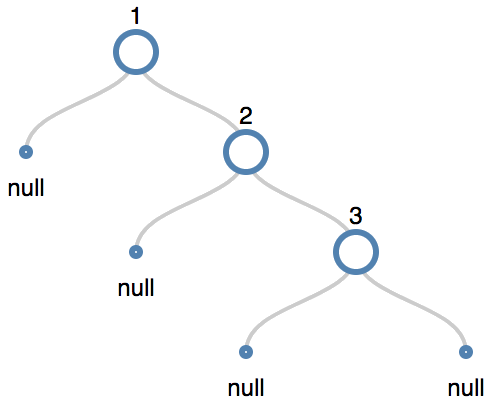We can add new child on the right that causes the root to become right-heavy like this:

We can fix this by rotating the tree left about 1.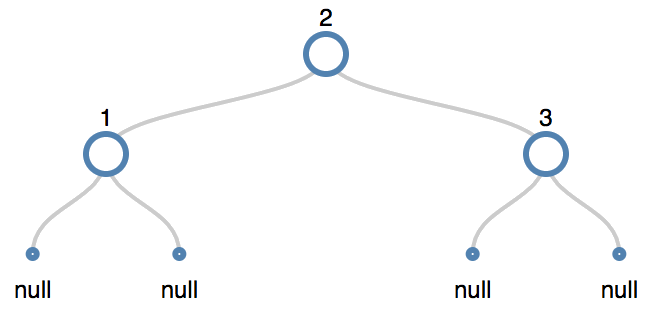Now the tree is balanced.

 Left side, left child.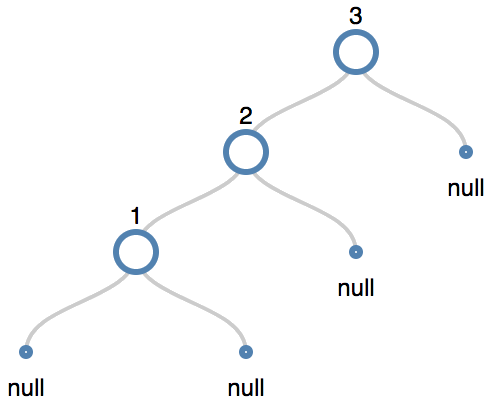We can fix this by rotating the tree right about 3.Note that when the node that caused the imbalance is on the same side of its parent as its parent is of its parent, we perform a rotation that is opposite the direction of this side about the parent’s parent to correct the behavior.

With numbers for the two cases, since that’s super confusing:

•  2 is on the right of its parent 1. 3 is also on the right of its parent 2. We fix the imbalance by rotating left (opposite of right) about the grandparent, 1.
•  is on the left side of its parent 3. 1 is also on the left side of its parent 2. We fix the imbalance by rotating right (opposite of left) about the grandparent, 3.

### Double Rotations Required

The other two cases require us to rotate twice. The first rotation reduces the problem to one of the two problems above.

Notably, the first in a double rotation is about the first child of the imbalancedd node, not the second.

 Right side, left child.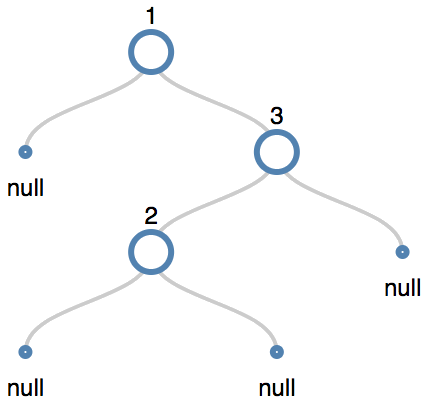First we have to rotate right about three, and then left about one.

Rotating right about three gives us:

``````1
\
2
\
3
``````

after which it is easy to create the balanced tree, applying rotation  from above.

 Left side, right child.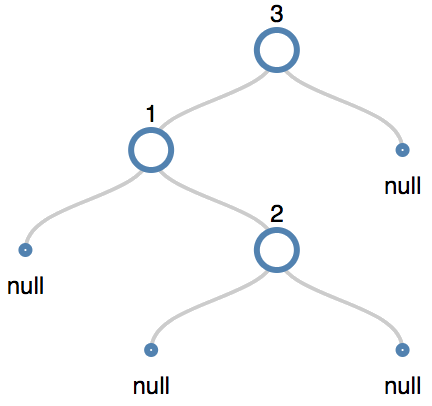First we have to rotate left about one, and then right about three.

Rotating left about one gives us:

``````    3
/
2
/
1
``````

after which it is easy to create the balanced tree by applying rotation  from above.

## Coding Strategy

When we insert an element, we’ll record the nodes that we traversed to get to the insertion spot in a stack. Then we’ll pop items off the stack one by one, retracing our steps to the tree root. At each node, we’ll recalculate its balance. If the node is imbalanced, we’ll apply the appropriate balancing rotations.

How do we rotate imbalanced nodes? We can use the sign of the imbalance to determine this.

Remember that Balance will be Left Branch Length - Right Branch Length. If the balance is -2, the node is right-heavy. If the balance is +2, the node is left-heavy.

If we encounter balance -2, we are either a ` Right side, right child` or ` Right side, left child` case. We can determine which by looking at the balance of the right child (we know right, since the parent is right-heavy) of this imbalanced parent.

The child will have balance of either 1 or -1.

If the balance is 1, we know that we are in case ` Right side, left child` and require a double rotation (child right-parent left).

If the balance is -1, we are in case ` Right side, right child` case and only require a single rotation (parent left).

BF Parent   BF Child   Rotations to Perform
2   -1   Child left, Parent right
-2   1   Child right, Parent left
2   1   Parent right
-2   -1   Parent left

## Code Implementation

We’ll perform a depth-first search to find the spot at which to insert the node.

For inserts, we can observe that since we recurse down the tree until we reach a null (a leaf node), we don’t have to worry about the inserted node itself being imbalanced. We only have to check its parent node and all other nodes on that path to the top of the tree.

To facilitate checking, we’ll store the nodes we traverse in a stack, since we are going from root to child to find the node, and we want to trace back from child to root to perform balancing. As we push items onto the stack, we’ll descend, and as we remove items from the top of the stack, we’ll ascend.

When we remove nodes from the stack, we check each one to see if it is balanced. We’ll write a simple method for the node, `getBF` that fetches the balance factor (the difference in the max height of the left subtree and the max height of the right subtree). If we encounter nodes whose difference is -2 or 2, we perform the appropriate rebalancing procedure.

``````node.prototype.getBF = function() {
return maxHeight(this.left)-maxHeight(this.right);
}
function maxHeight(tree) {
if (tree==null) { return 0; }
else {
let leftD=maxHeight(tree.left);
let rightD=maxHeight(tree.right);
return leftD > rightD ? leftD+1 : rightD+1;
}
}
``````

We’ll use our previous node search method, adding a stack so that if we find the insert point, we can retrace our path up the tree and correct any imbalances.

Here’s what it looks like.

``````function balanceNode(q, tree) { //balances a node
let bf=q.getBF();
if (bf==2||bf==-2) {
if (bf==2) { //left-heavy parent
let childBalance=q.left.getBF();
if (childBalance==1) { //left-child
rotateRight(tree, q);
} else if (childBalance==-1) { //right child
rotateLeft(tree, q.left);
rotateRight(tree, q);
}
}
else if (bf==-2) { //right-heavy parent
let childBalance=q.right.getBF();
if (childBalance==1) { //left-child
rotateLeft(tree, q);
} else if (childBalance==-1) { //right child
rotateRight(tree, q.right);
rotateLeft(tree, q);
}
}
}
}
``````

Things are a little bit terse, but think about what’s happening here. For a given node, we check its balance factor. If the node has a balance factor of -2 or 2, it’s imbalanced. We then look to either the left (2) or right (-2) child, depending on balance factor. We check to see which direction that child is imbalanced in, either (1) left or (-1) right.

Then, all we do is look up the balance factor values in the chart we prepared and code in the appropriate functions.

BF Parent   BF Child   Rotations to Perform
2   -1   Child left, Parent right
-2   1   Child right, Parent left
2   1   Parent right
-2   -1   Parent left

Super easy. That’s literally all there is to insertions.

## Congratulations

You now understand the basics of insertions into a balanced binary tree. By performing rebalancing operations, the tree become more performant in lookup. No longer will it degenerate to a linked list (just try adding 1, 2, 3, 4, 5, 6, 7, 8, 9, 10… in the CodePen and watch how the tree responds).

Next time we’ll cover deletion. It’s not too tricky, but let’s pace ourselves.